# Simple harmonic motion Questions and Answers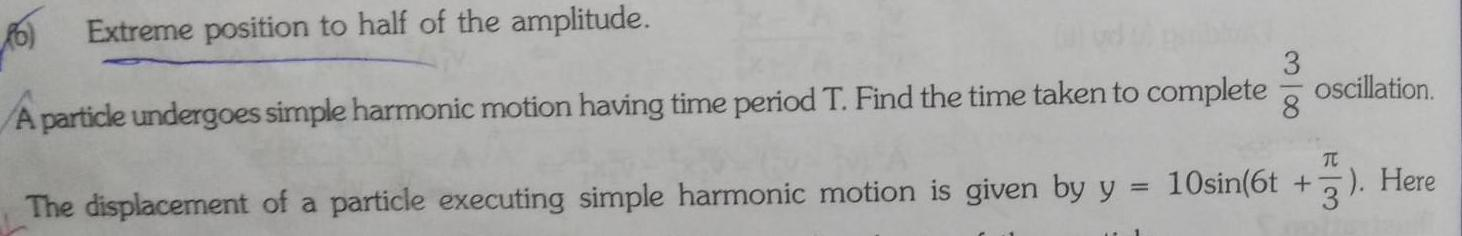Physics
Simple harmonic motion
6 Extreme position to half of the amplitude A particle undergoes simple harmonic motion having time period T Find the time taken to complete 318 oscillation TC The displacement of a particle executing simple harmonic motion is given by y 10sin 6t 3 Here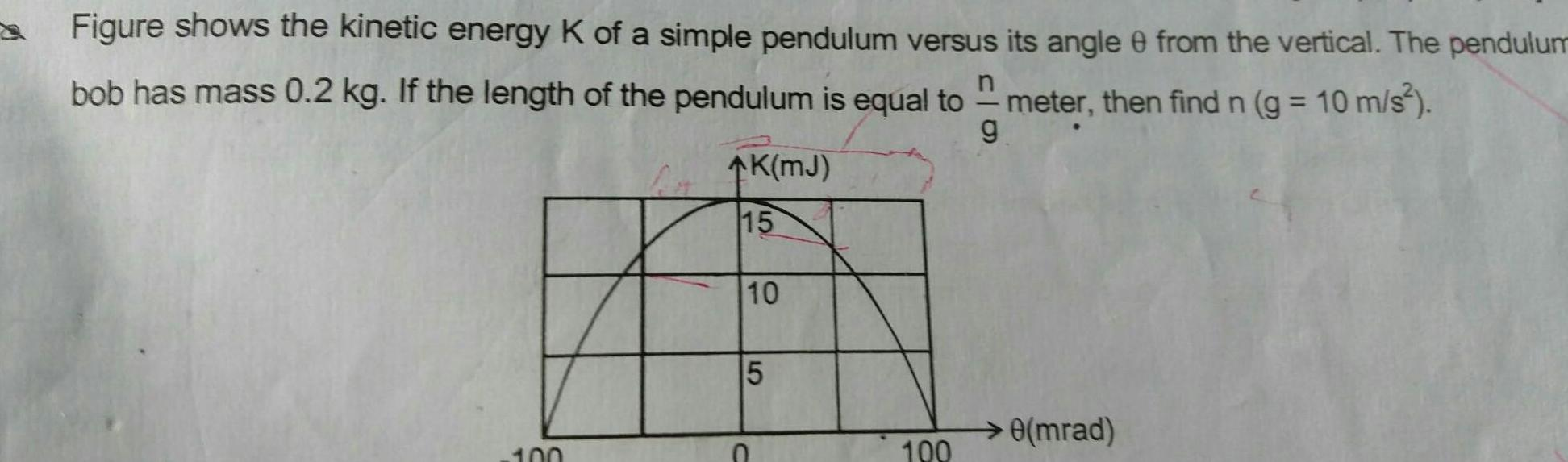Physics
Simple harmonic motion
Figure shows the kinetic energy K of a simple pendulum versus its angle 0 from the vertical The pendulum bob has mass 0 2 kg If the length of the pendulum is equal to g meter then find n g 10 m s 100 K MJ 15 10 5 100 0 mrad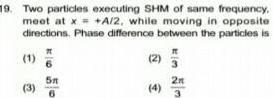Physics
Simple harmonic motion
19 Two particles executing SHM of same frequency meet at x A 2 while moving in opposite directions Phase difference between the particles is It 1 3 51 2 4 2 3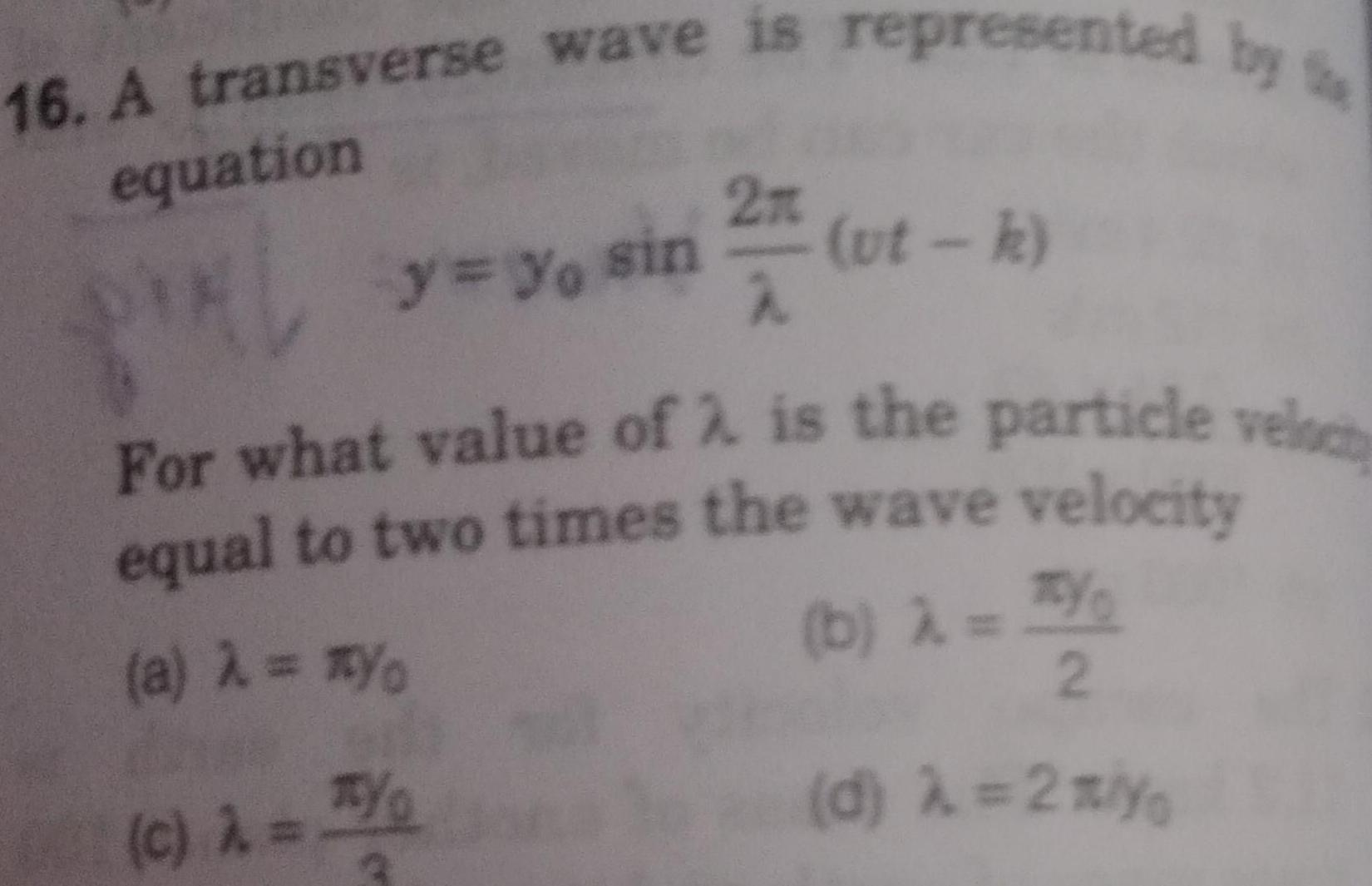Physics
Simple harmonic motion
16 A transverse wave is represented by equation y yo sin 32 74 ut k For what value of 2 is the particle veloc equal to two times the wave velocity a Yo b c TY 2 d 2 yo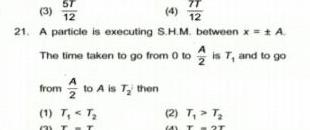Physics
Simple harmonic motion
ST 3 4 12 12 21 A particle is executing S H M between x A The time taken to go from 0 to is T and to go from to A is T then 1 T T T 2 T T T 2T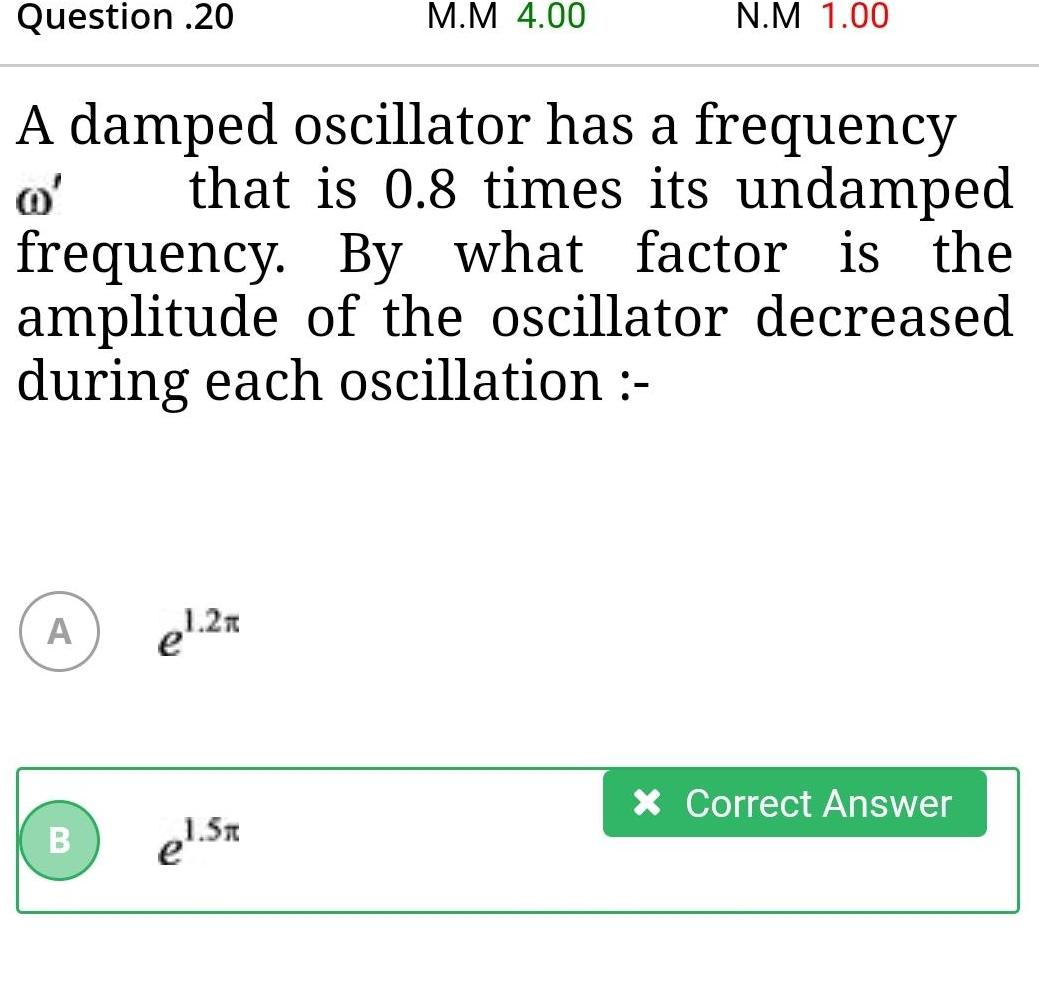Physics
Simple harmonic motion
Question 20 A A damped oscillator has a frequency that is 0 8 times its undamped frequency By what factor is the amplitude of the oscillator decreased during each oscillation B el 2x M M 4 00 1 5x N M 1 00 Correct Answer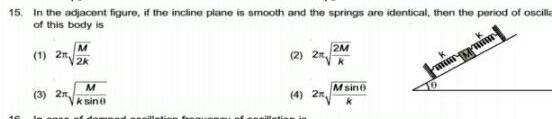Physics
Simple harmonic motion
15 In the adjacent figure if the incline plane is smooth and the springs are identical then the period of oscilla of this body is 1 2n 3 2 M 2k M ksine 2 4 2 2M Msin 10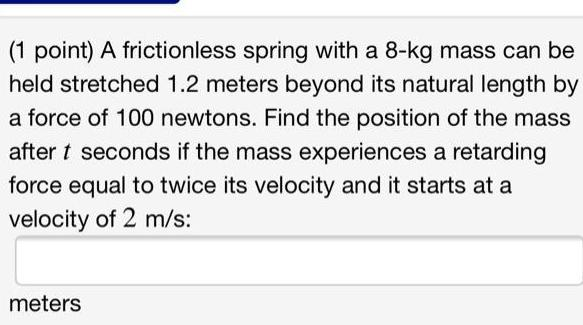Physics
Simple harmonic motion
1 point A frictionless spring with a 8 kg mass can be held stretched 1 2 meters beyond its natural length by a force of 100 newtons Find the position of the mass after t seconds if the mass experiences a retarding force equal to twice its velocity and it starts at a velocity of 2 m s meters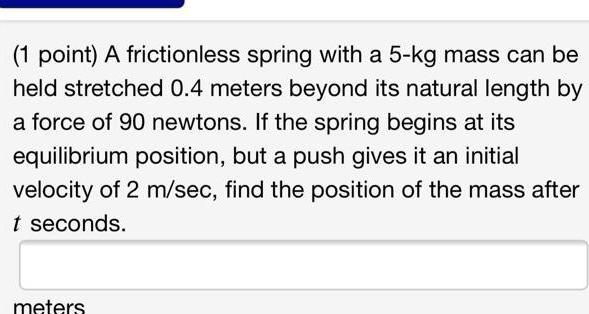Physics
Simple harmonic motion
1 point A frictionless spring with a 5 kg mass can be held stretched 0 4 meters beyond its natural length by a force of 90 newtons If the spring begins at its equilibrium position but a push gives it an initial velocity of 2 m sec find the position of the mass after t seconds meters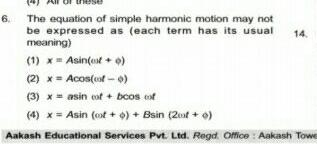Physics
Simple harmonic motion
6 The equation of simple harmonic motion may not be expressed as each term has its usual 14 meaning 1 x Asin et o 2 x Acos oof o 3 xasin cof bcos cof 4 x Asin oot o Bsin 2 0 Aakash Educational Services Pvt Ltd Regd Office Aakash Towe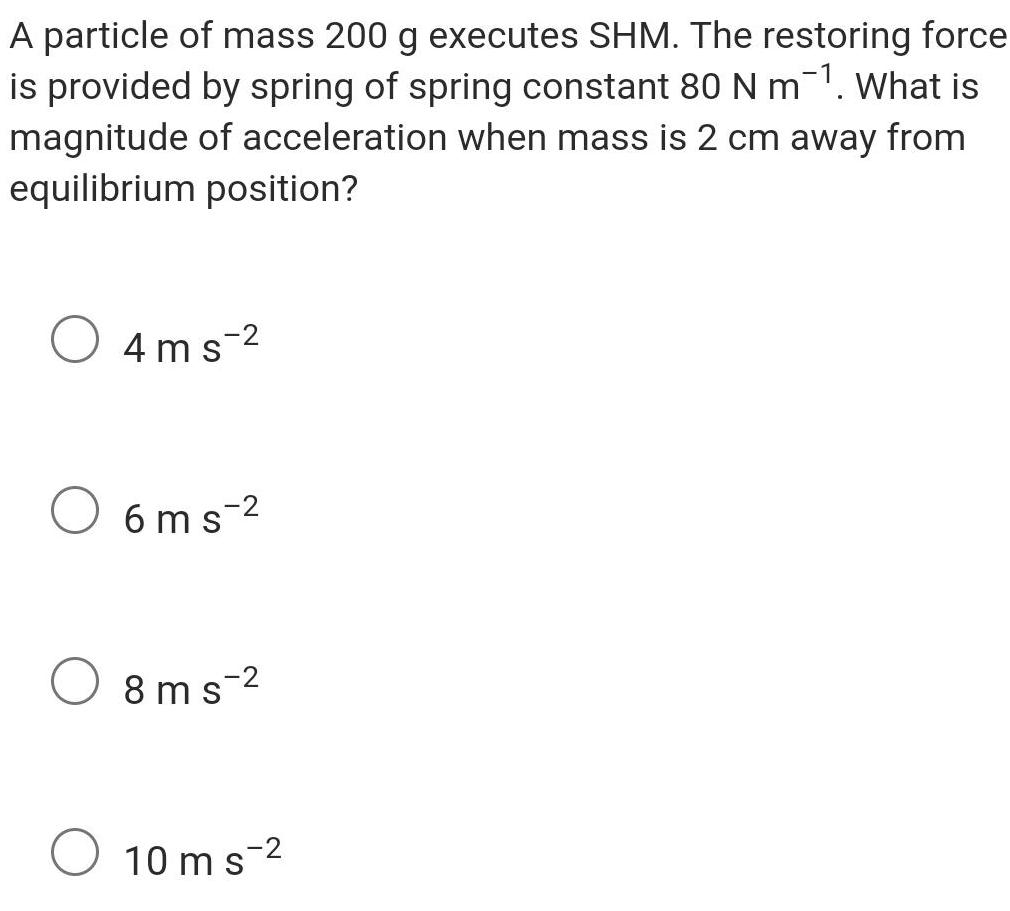Physics
Simple harmonic motion
A particle of mass 200 g executes SHM The restoring force is provided by spring of spring constant 80 N m What is magnitude of acceleration when mass is 2 cm away from equilibrium position 4ms 2 6ms 8ms 2 10 ms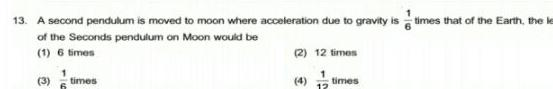Physics
Simple harmonic motion
13 A second pendulum is moved to moon where acceleration due to gravity is times that of the Earth the le of the Seconds pendulum on Moon would be 1 6 times 2 12 times 3 times 12 times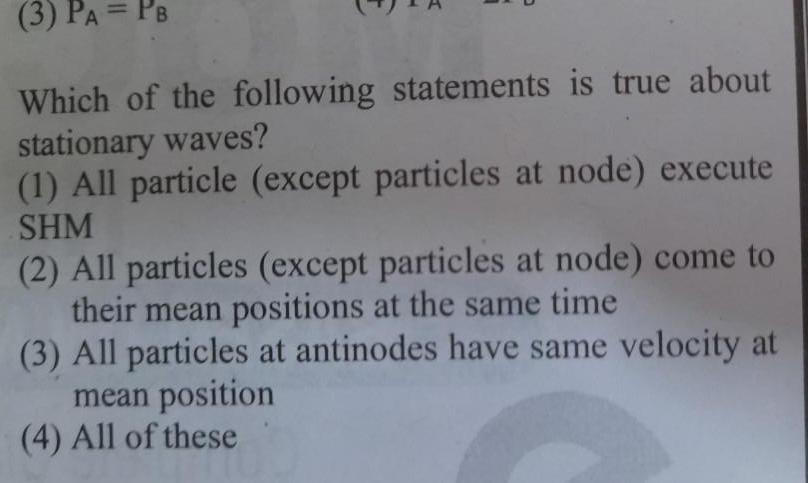Physics
Simple harmonic motion
3 PA PB Which of the following statements is true about stationary waves 1 All particle except particles at node execute SHM 2 All particles except particles at node come to their mean positions at the same time 3 All particles at antinodes have same velocity at mean position 4 All of these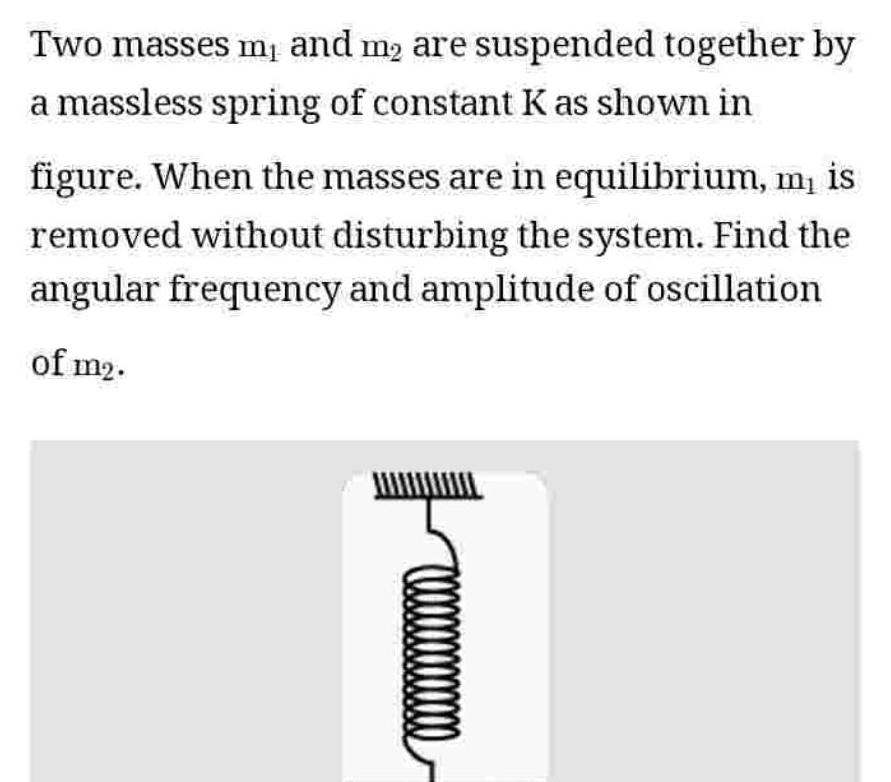Physics
Simple harmonic motion
Two masses m and m are suspended together by a massless spring of constant K as shown in figure When the masses are in equilibrium m is removed without disturbing the system Find the angular frequency and amplitude of oscillation of m2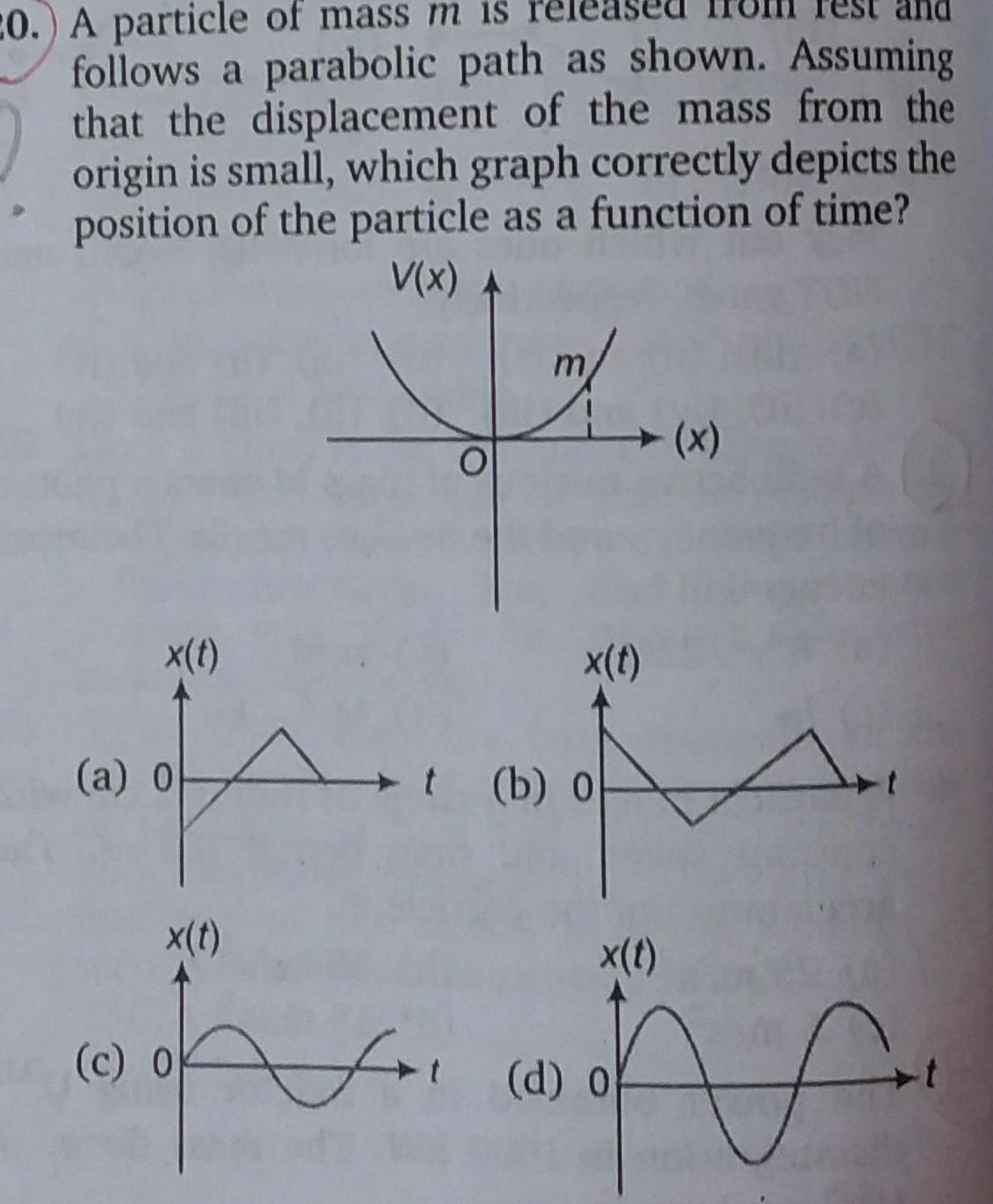Physics
Simple harmonic motion
20 A particle of mass m is released follows a parabolic path as shown Assuming that the displacement of the mass from the origin is small which graph correctly depicts the position of the particle as a function of time V x x t a o x t c 0 O x t b o x x t d o for t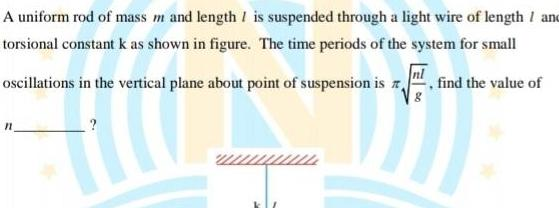Physics
Simple harmonic motion
A uniform rod of mass m and length I is suspended through a light wire of length and torsional constant k as shown in figure The time periods of the system for small oscillations in the vertical plane about point of suspension is find the value of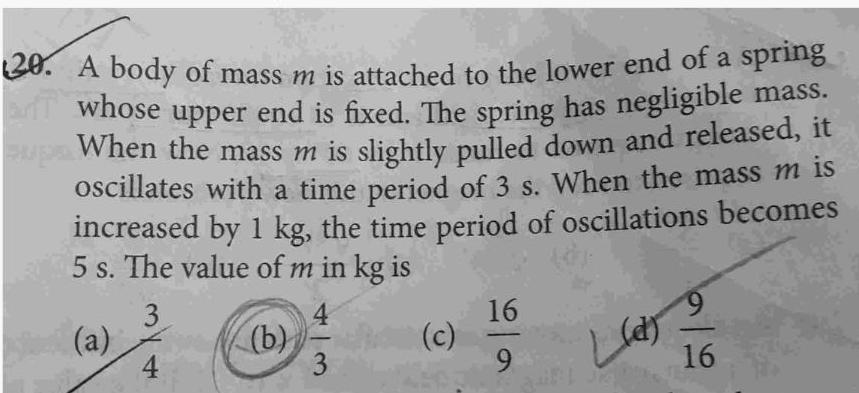Physics
Simple harmonic motion
20 A body of mass m is attached to the lower end of a spring an whose upper end is fixed The spring has negligible mass supe When the mass m is slightly pulled down and released it oscillates with a time period of 3 s When the mass m is increased by 1 kg the time period of oscillations becomes 5 s The value of m in kg is 3 a 4 b 4 3 c 16 9 9 Lun 22 16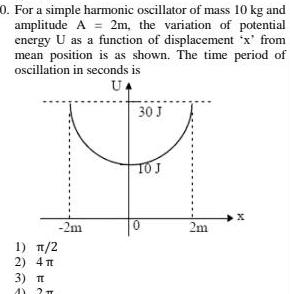Physics
Simple harmonic motion
0 For a simple harmonic oscillator of mass 10 kg and amplitude A 2m the variation of potential energy U as a function of displacement x from mean position is as shown The time period of oscillation in seconds is UA 1 2 2 4 3 T AY 2 T 2m 30 J TO J 0 2m X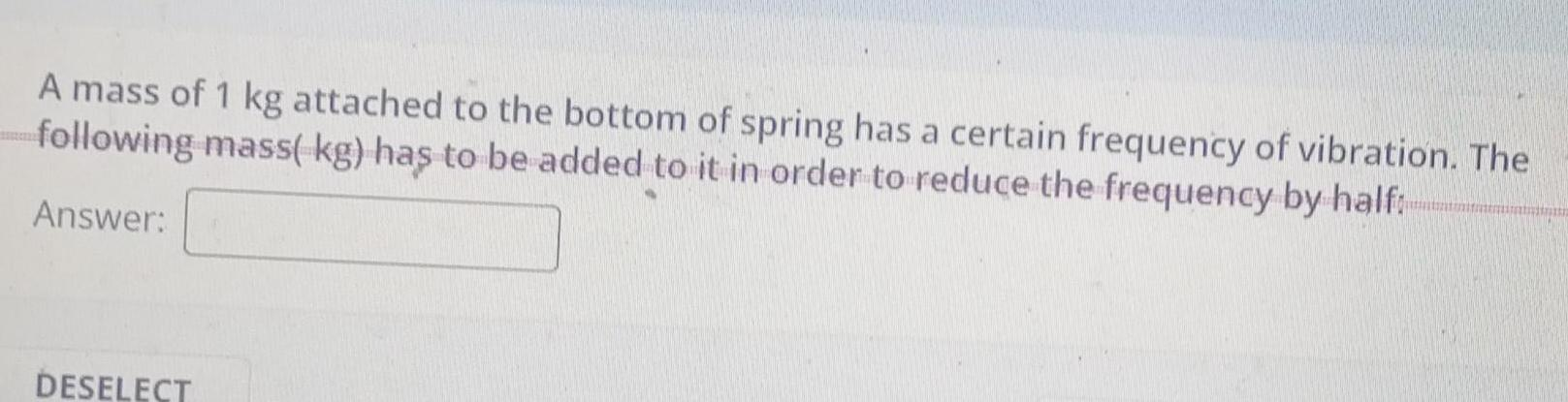Physics
Simple harmonic motion
A mass of 1 kg attached to the bottom of spring has a certain frequency of vibration The following mass kg has to be added to it in order to reduce the frequency by half Answer DESELECT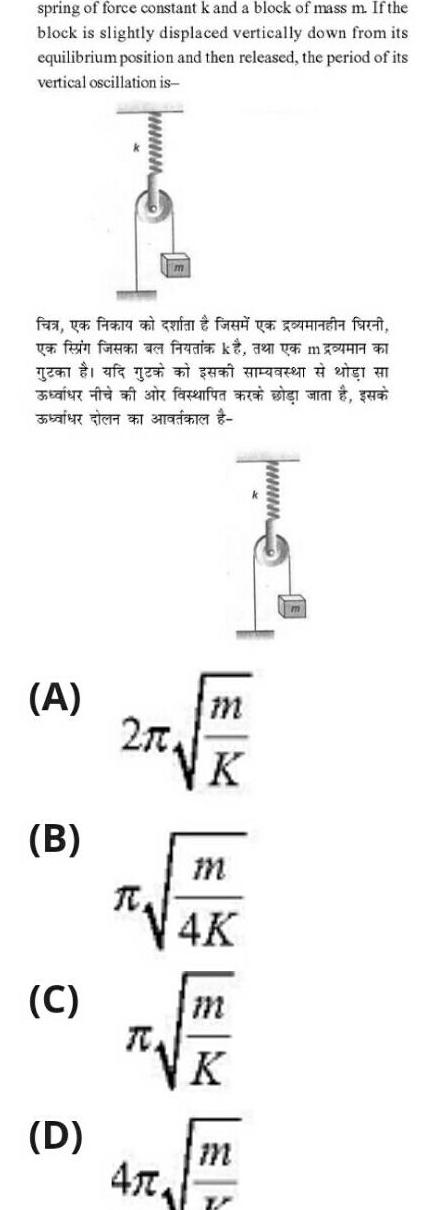Physics
Simple harmonic motion
spring of force constant k and a block of mass m If the block is slightly displaced vertically down from its equilibrium position and then released the period of its vertical oscillation is fea ya fiera an quan fad ya una farci yen fein foren a gen af g a A B C D facenfura a visi m 2 0 K R 47 m 4K K m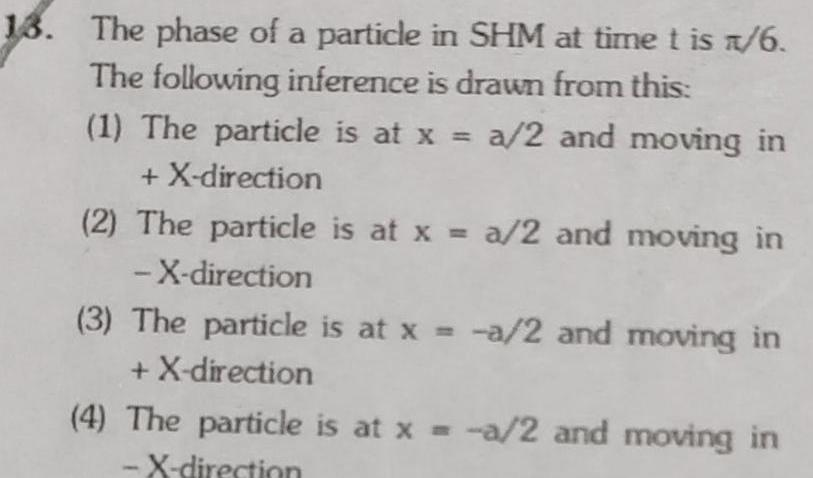Physics
Simple harmonic motion
13 The phase of a particle in SHM at time t is 1 6 The following inference is drawn from this 1 The particle is at x a 2 and moving in X direction 2 The particle is at x a 2 and moving in X direction 3 The particle is at x a 2 and moving in X direction 4 The particle is at x a 2 and moving in X direction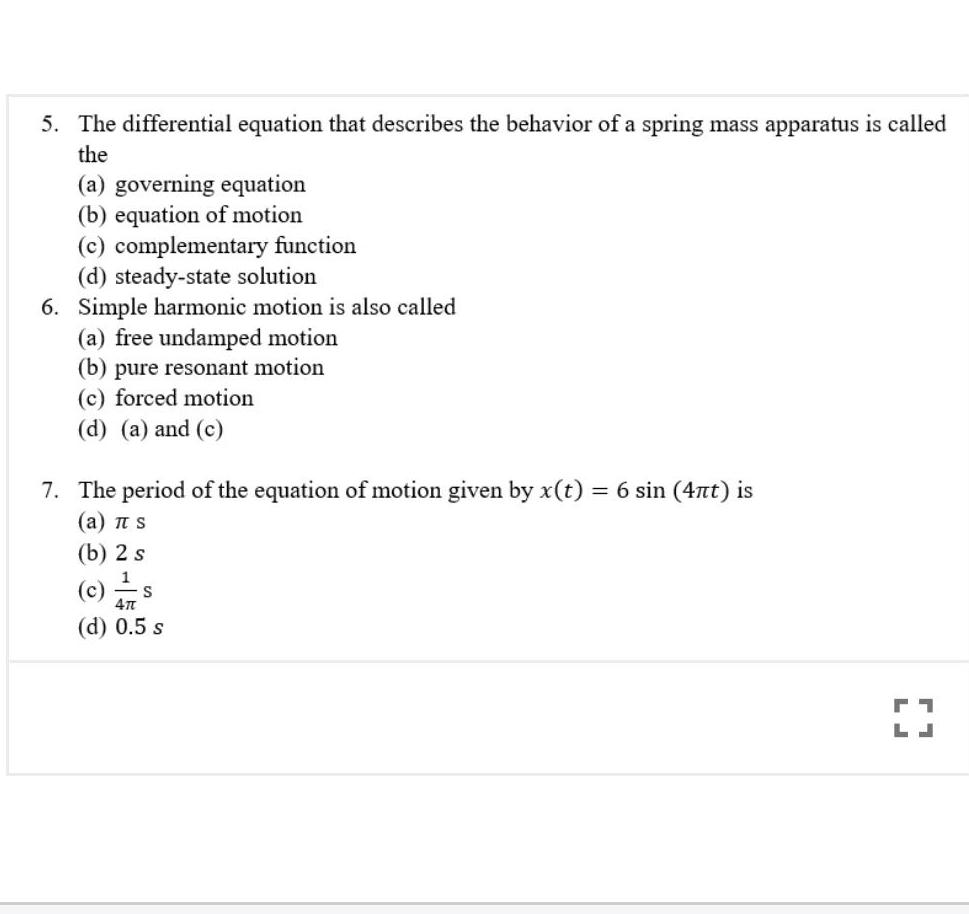Physics
Simple harmonic motion
5 The differential equation that describes the behavior of a spring mass apparatus is called the a governing equation b equation of motion c complementary function d steady state solution 6 Simple harmonic motion is also called a free undamped motion b pure resonant motion c forced motion d a and c 7 The period of the equation of motion given by x t 6 sin 4nt is a TT S b 2 s 1 c 47 d 0 5 s S 71 LJ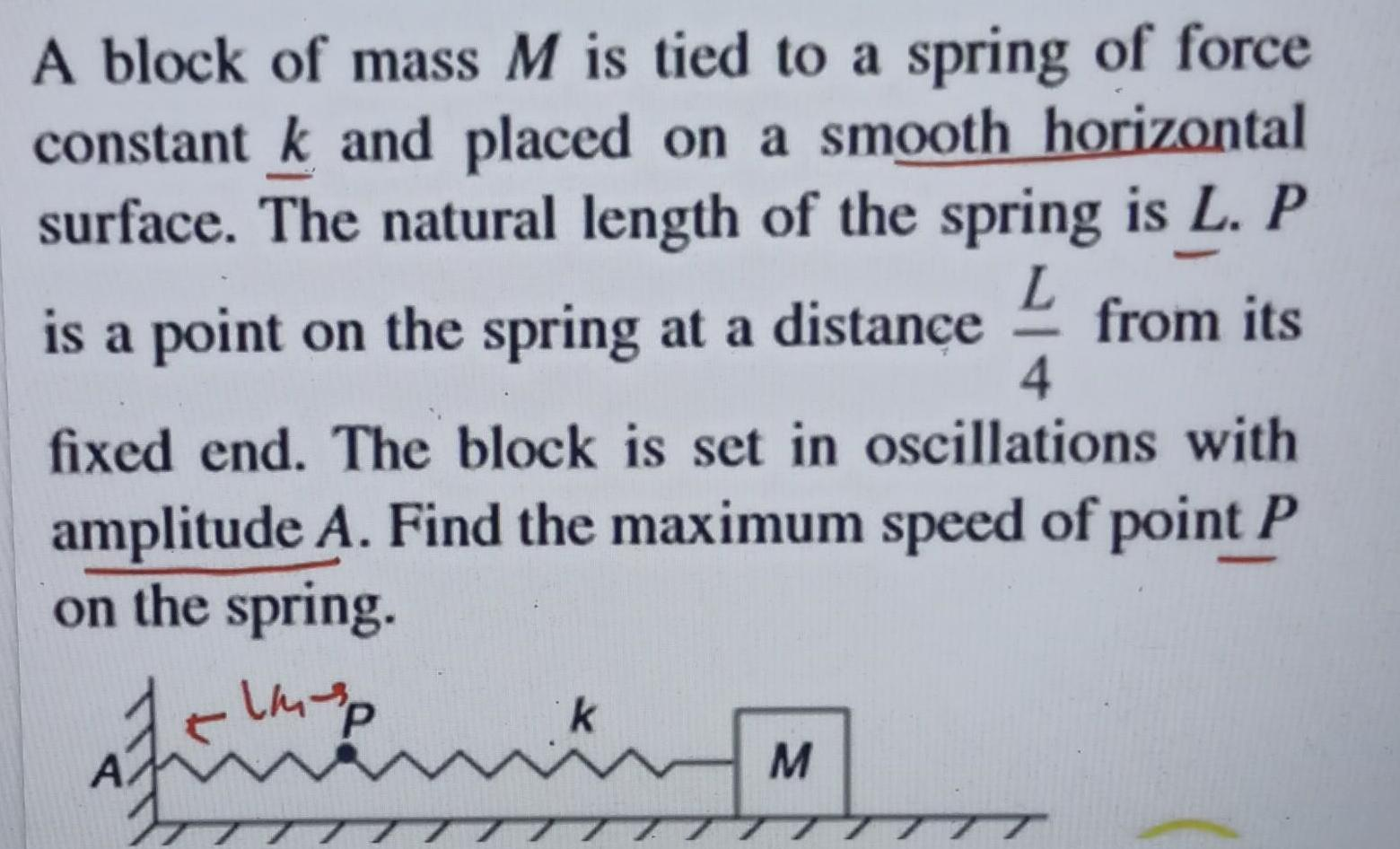Physics
Simple harmonic motion
L A block of mass M is tied to a spring of force constant k and placed on a smooth horizontal surface The natural length of the spring is L P is a point on the spring at a distance from its fixed end The block is set in oscillations with amplitude A Find the maximum speed of point P on the spring 4 atau yang g k M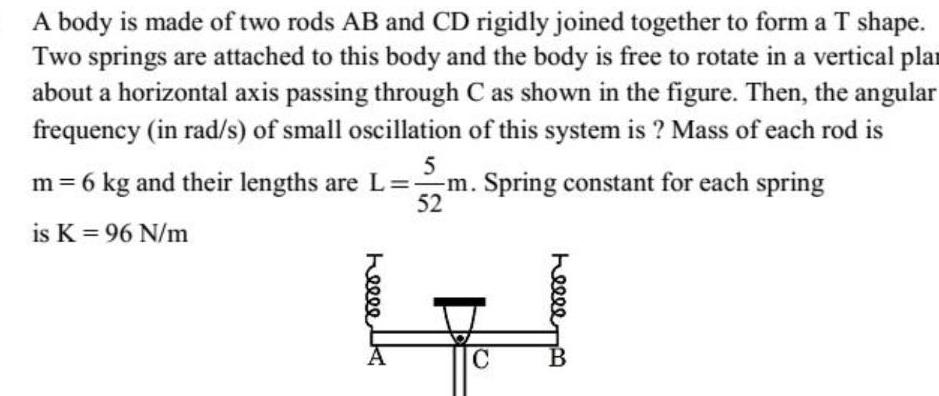Physics
Simple harmonic motion
A body is made of two rods AB and CD rigidly joined together to form a T shape Two springs are attached to this body and the body is free to rotate in a vertical pla about a horizontal axis passing through C as shown in the figure Then the angular frequency in rad s of small oscillation of this system is Mass of each rod is 5 m 6 kg and their lengths are L m Spring constant for each spring is K 96 N m 52 helle K C helle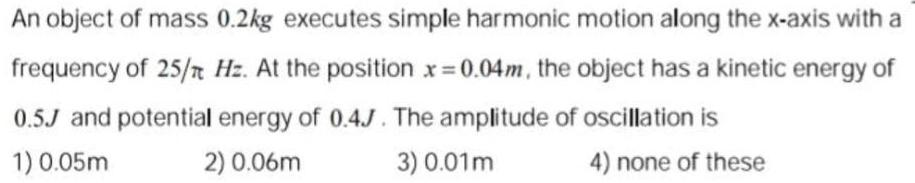Physics
Simple harmonic motion
An object of mass 0 2kg executes simple harmonic motion along the x axis with a frequency of 25 Hz At the position x 0 04m the object has a kinetic energy of 0 5J and potential energy of 0 4J The amplitude of oscillation is 1 0 05m 2 0 06m 3 0 01m 4 none of these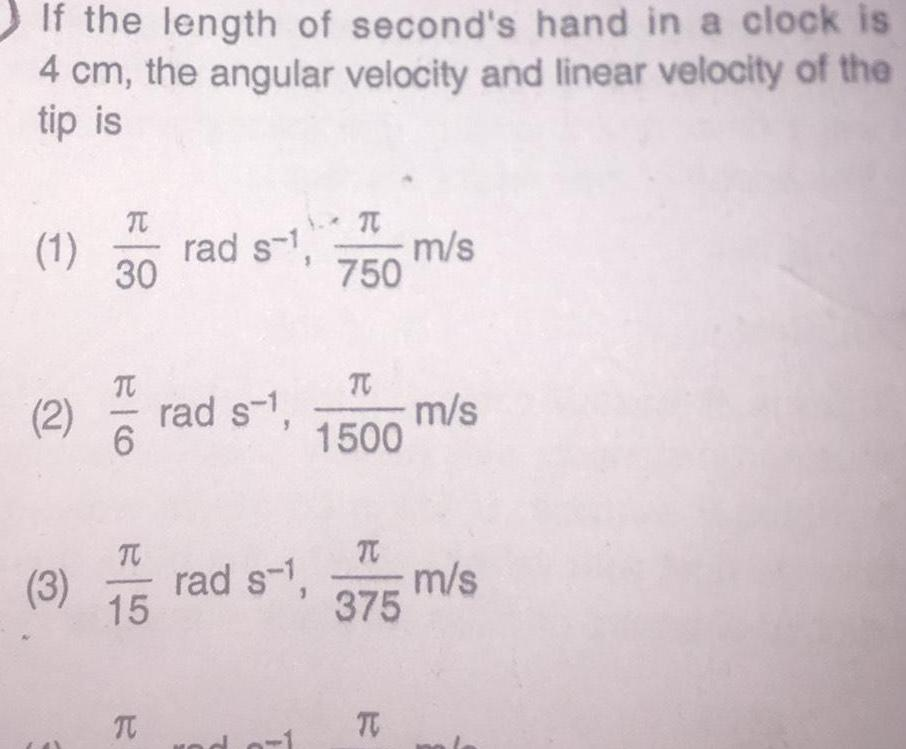Physics
Simple harmonic motion
If the length of second s hand in a clock is 4 cm the angular velocity and linear velocity of the tip is T 1 rad s 1 30 1 2 3 6 15 T rad s 1 T rad s C 750 TC 1500 T 375 T m s m s m s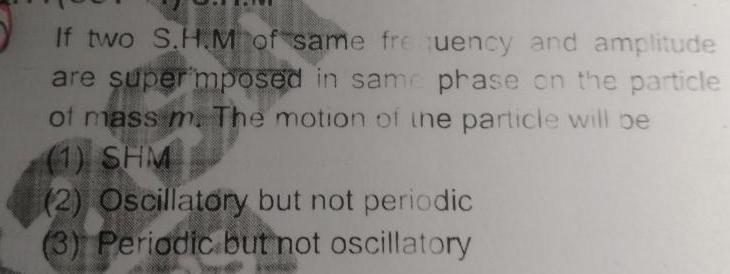Physics
Simple harmonic motion
If two S H M of same frequency and amplitude are super mposed in same phase on the particle of mass m The motion of the particle will be 1 SHM 2 Oscillatory but not periodic 3 Periodic but not oscillatory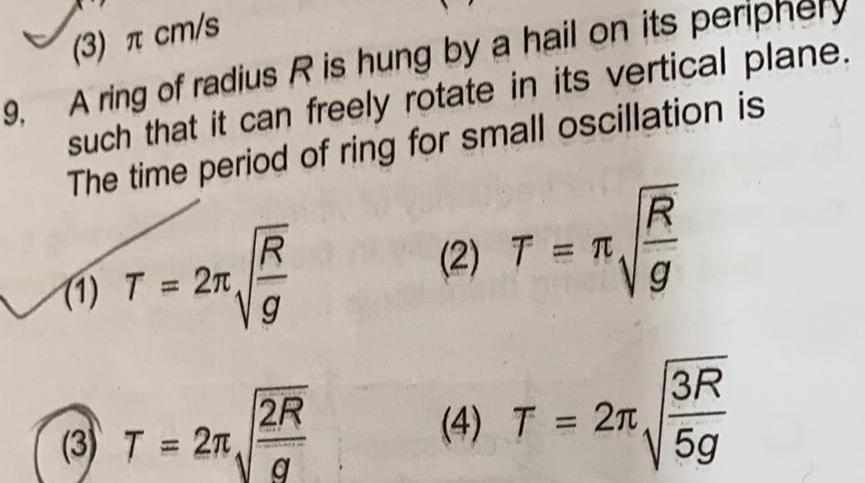Physics
Simple harmonic motion
3 cm s 9 A ring of radius R is hung by a hail on its periphery such that it can freely rotate in its vertical plane The time period of ring for small oscillation is 1 T 2n 3 T 2T R g 2R g 2 T T R g 3R 5g 4 T 2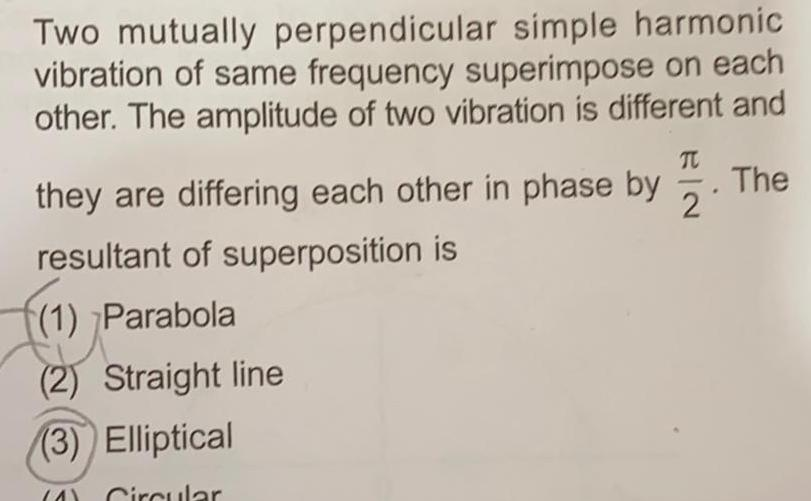Physics
Simple harmonic motion
Two mutually perpendicular simple harmonic vibration of same frequency superimpose on each other The amplitude of two vibration is different and T they are differing each other in phase by resultant of superposition is 1 Parabola 2 Straight line 3 Elliptical A Circular The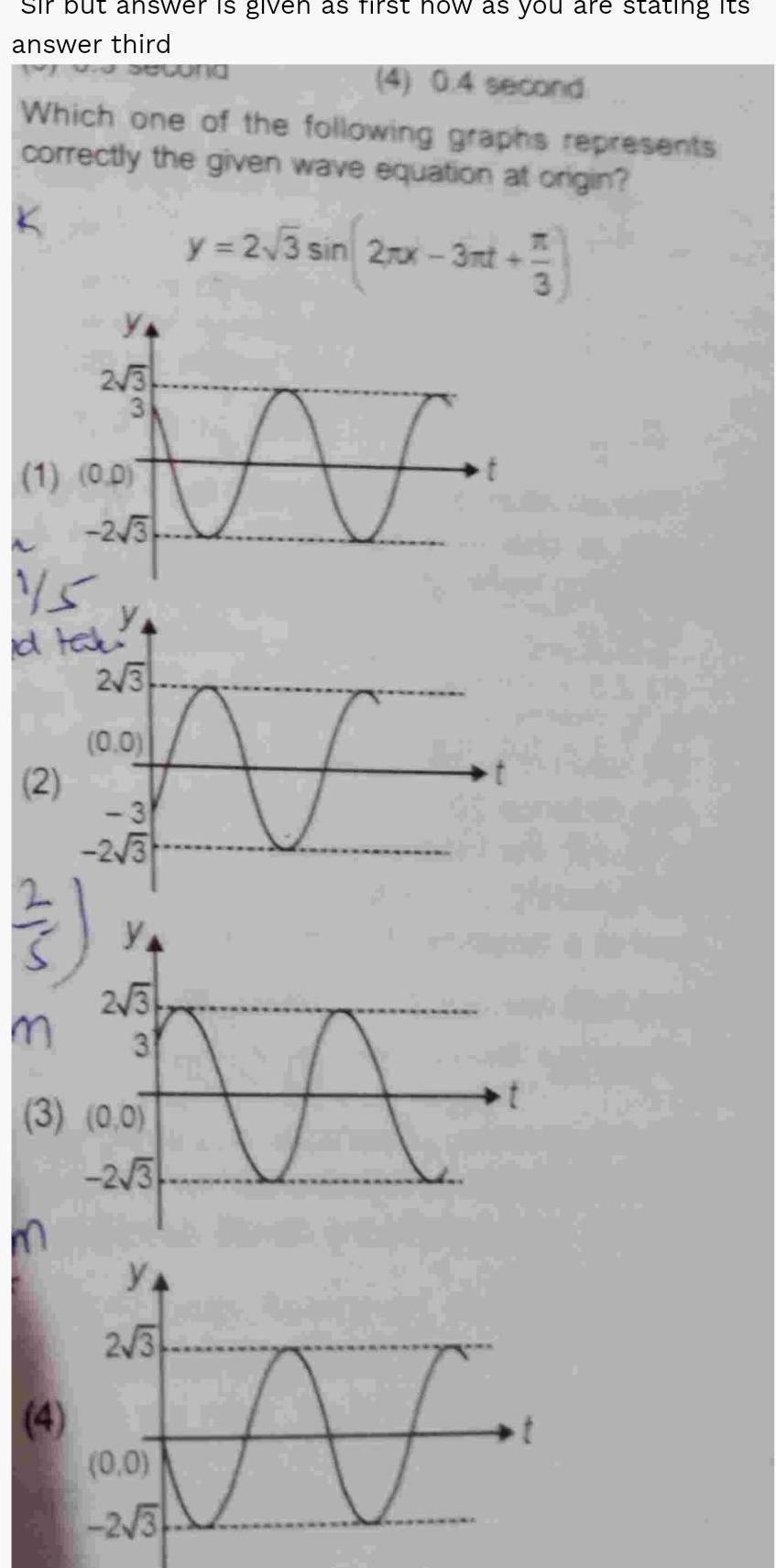Physics
Simple harmonic motion
Sir but answer is given as first how as you are stating its answer third 4 0 4 second Which one of the following graphs represents correctly the given wave equation at origin K 2 3 1 0 0 2 1S id tak m 2 3 N F 2 3 0 0 3 2 3 133 2 3 3 3 0 0 2 3 2 3 una y 2 3 sin 2rx 3mt 3 0 0 2 3 t N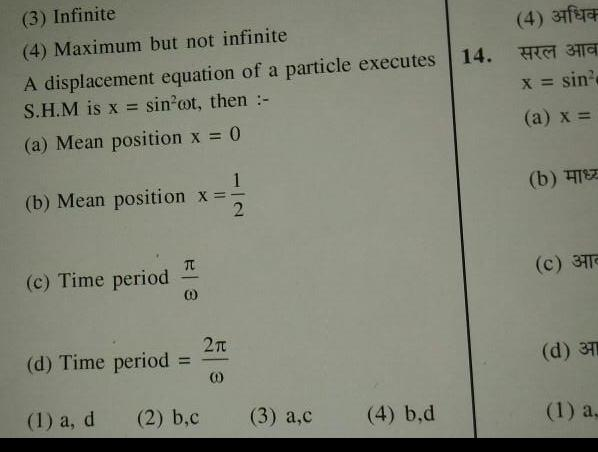Physics
Simple harmonic motion
3 Infinite 4 Maximum but not infinite A displacement equation of a particle executes S H M is x sin oot then a Mean position x 0 1 b Mean position x 2 c Time period 3 1 a d 0 d Time period 2 b c 2 0 3 a c 4 b d 14 4 37 x sin a x b c 371 d 37 1 a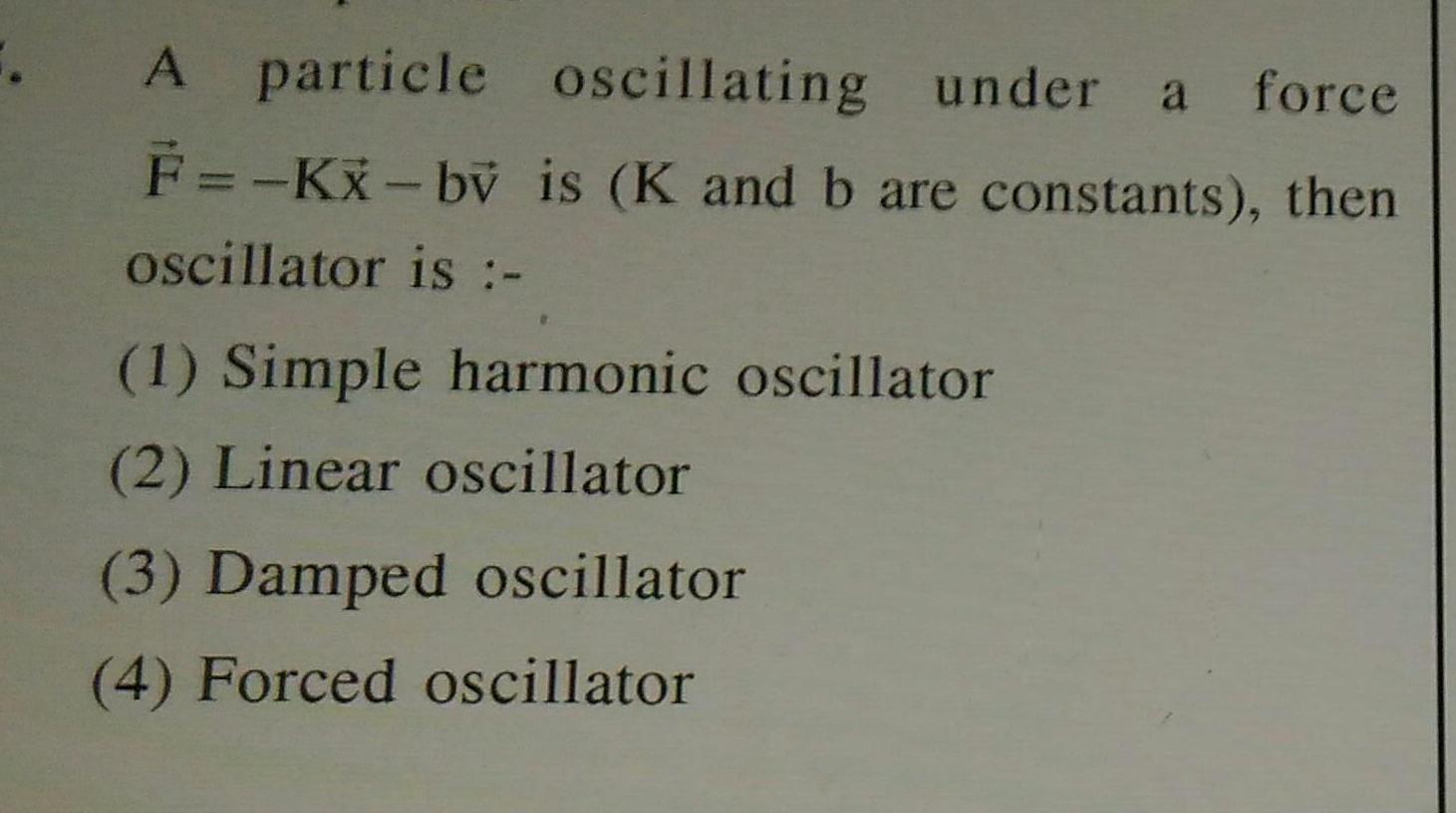Physics
Simple harmonic motion
A particle oscillating under a force F KX bv is K and b are constants then oscillator is 1 Simple harmonic oscillator 2 Linear oscillator 3 Damped oscillator 4 Forced oscillator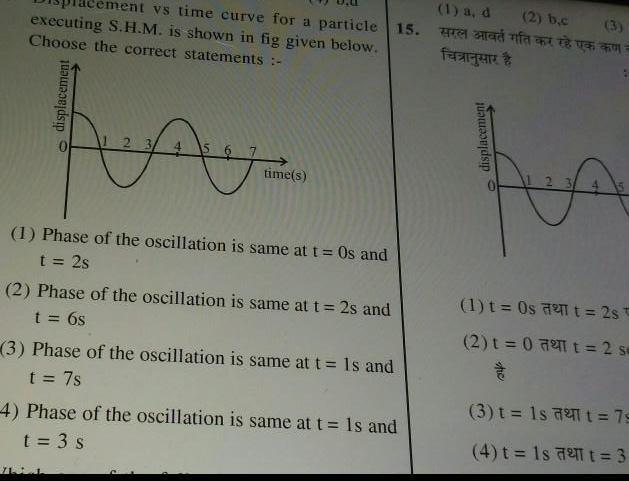Physics
Simple harmonic motion
executing S H M is shown in fig given below Choose the correct statements vs time curve for a particle 15 displacement Ay 1 Phase of the oscillation is same at t Os and t 2s time s 2 Phase of the oscillation is same at t 2s and t 6s 711 3 Phase of the oscillation is same at t Is and t 7s 4 Phase of the oscillation is same at t Is and t 3 s 1 a d feeigen displacement 2 b c 3 pa 1 t 0s 2 t 2st 2 t 0 72t 2s 3 t 1s 72 t 79 4 t 1s 72 t 3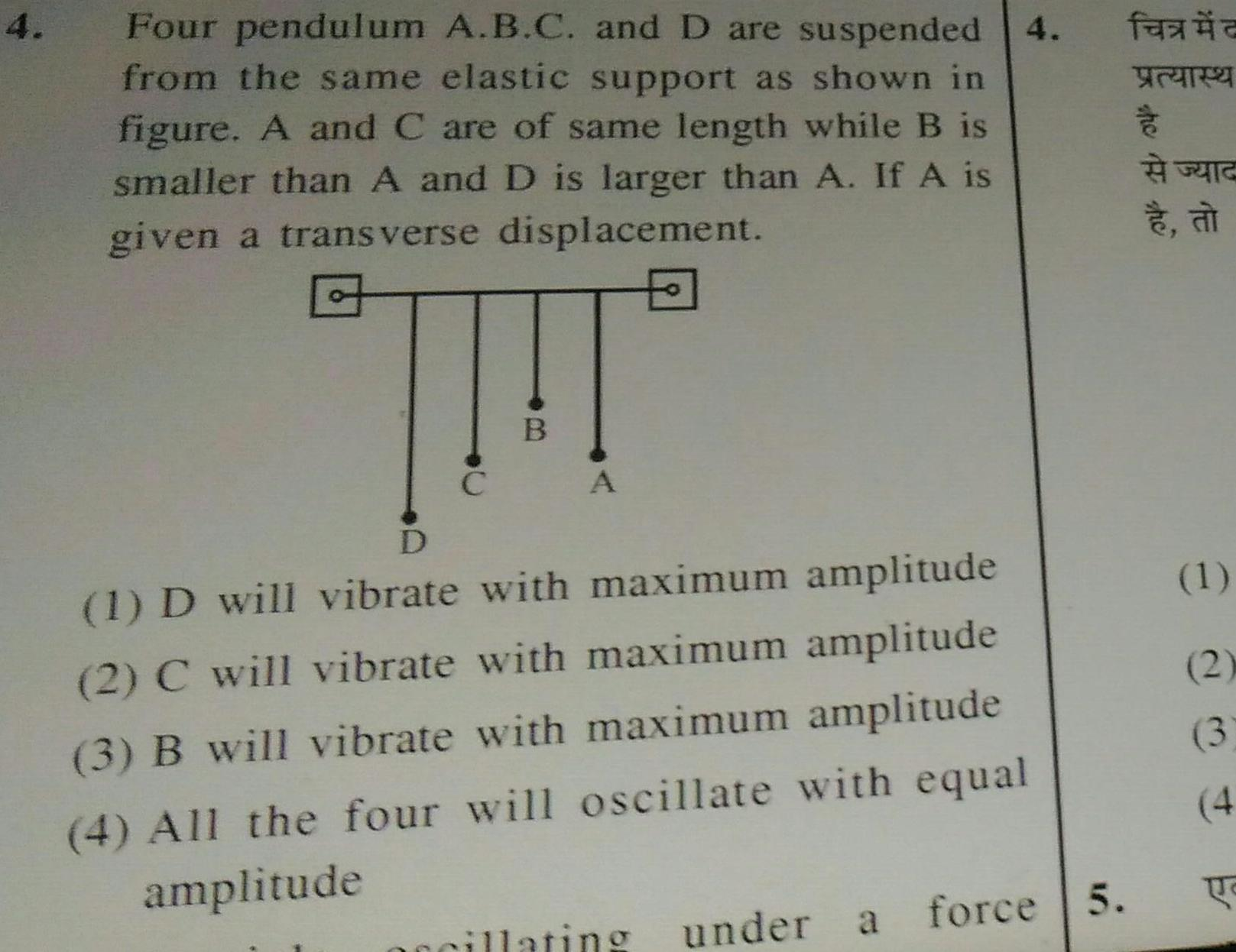Physics
Simple harmonic motion
4 Four pendulum A B C and D are suspended from the same elastic support as shown in figure A and C are of same length while B is smaller than A and D is larger than A If A is given a transverse displacement C B A D 1 D will vibrate with maximum amplitude 2 C will vibrate with maximum amplitude 3 B will vibrate with maximum amplitude 4 All the four will oscillate with equal amplitude accillating under a force 5 f FAR a 1 2 3 4 U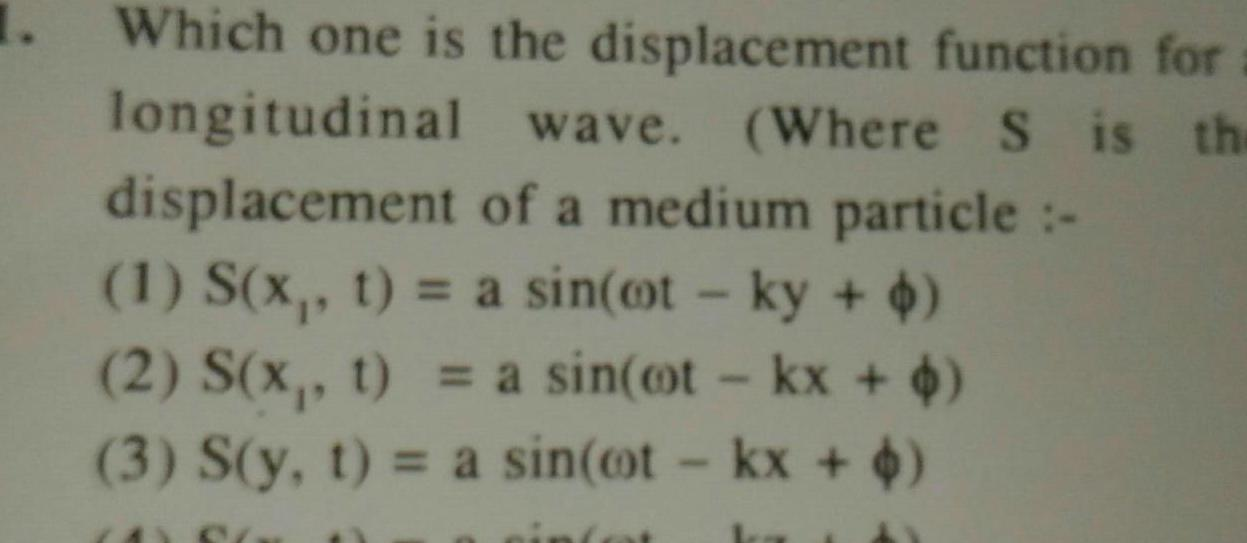Physics
Simple harmonic motion
Which one is the displacement function for wave Where S is the longitudinal displacement 1 S x t a sin ot ky 2 S x t a sin oot kx 4 3 S y t a sin cot kx 4 of a medium particle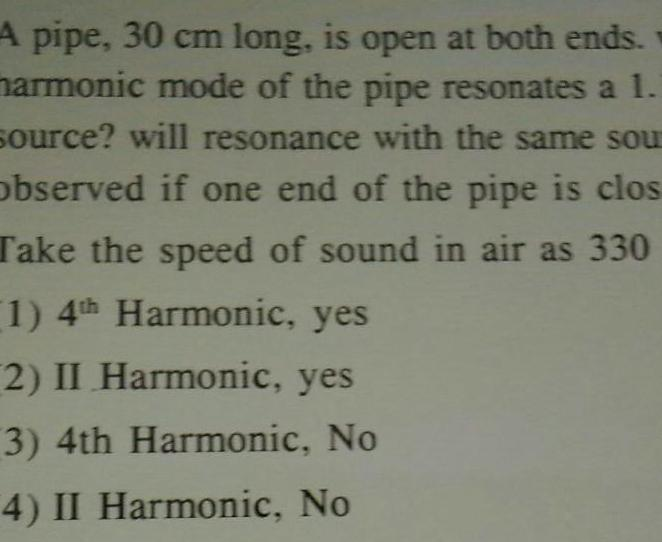Physics
Simple harmonic motion
A pipe 30 cm long is open at both ends harmonic mode of the pipe resonates a 1 source will resonance with the same sou observed if one end of the pipe is clos Take the speed of sound in air as 330 1 4th Harmonic yes 2 II Harmonic yes 3 4th Harmonic No 4 II Harmonic No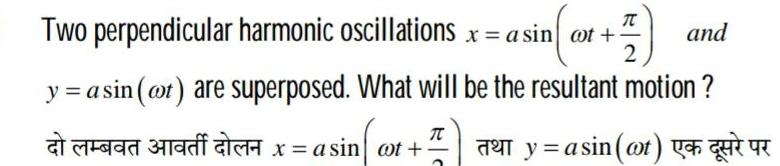Physics
Simple harmonic motion
T Two perpendicular harmonic oscillations x a sin oot 2 y a sin at are superposed What will be the resultant motion y sin at T add af x asin at and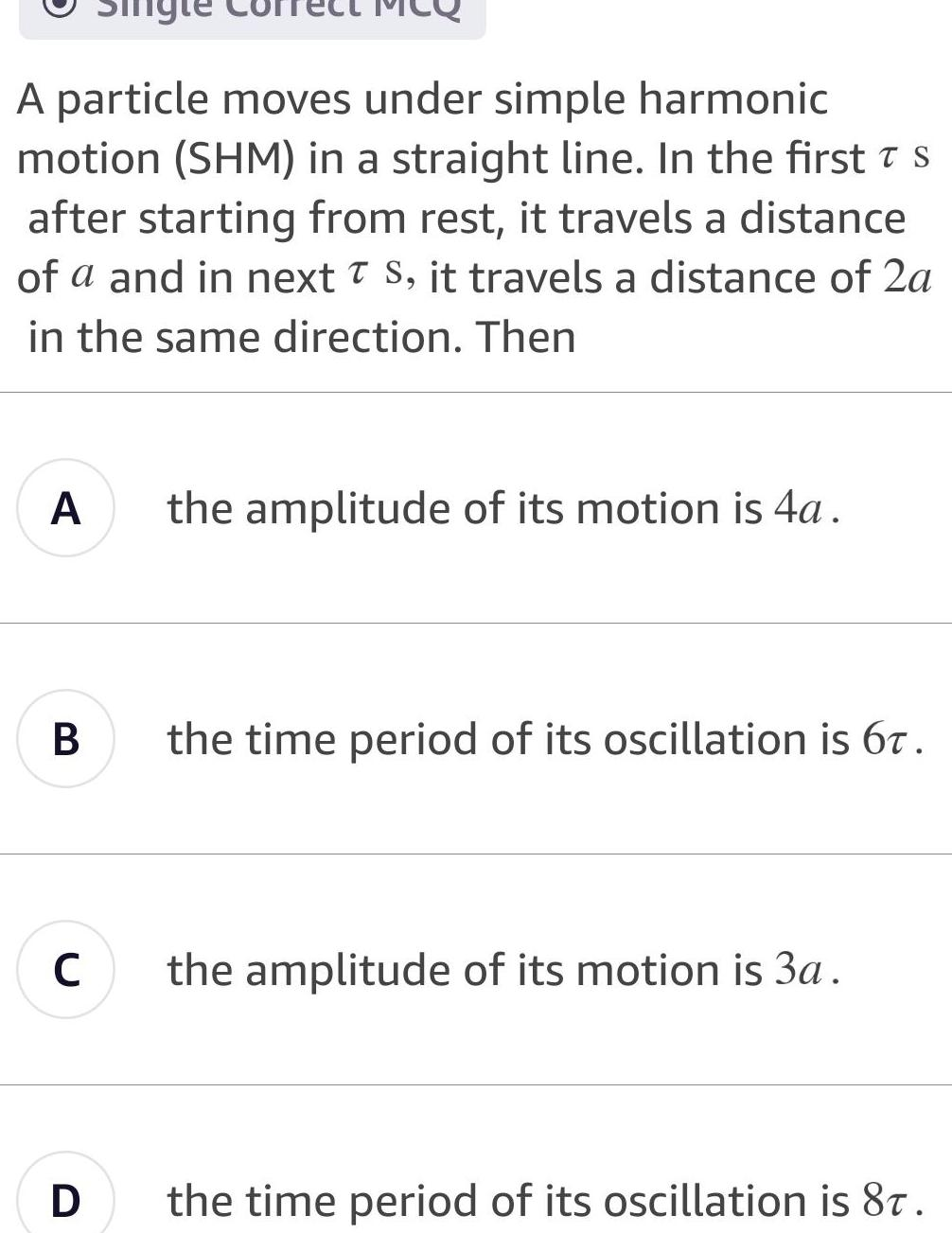Physics
Simple harmonic motion
A particle moves under simple harmonic motion SHM in a straight line In the first s after starting from rest it travels a distance of a and in next T S it travels a distance of 2a in the same direction Then A B C D the amplitude of its motion is 4a the time period of its oscillation is 67 the amplitude of its motion is 3a the time period of its oscillation is 87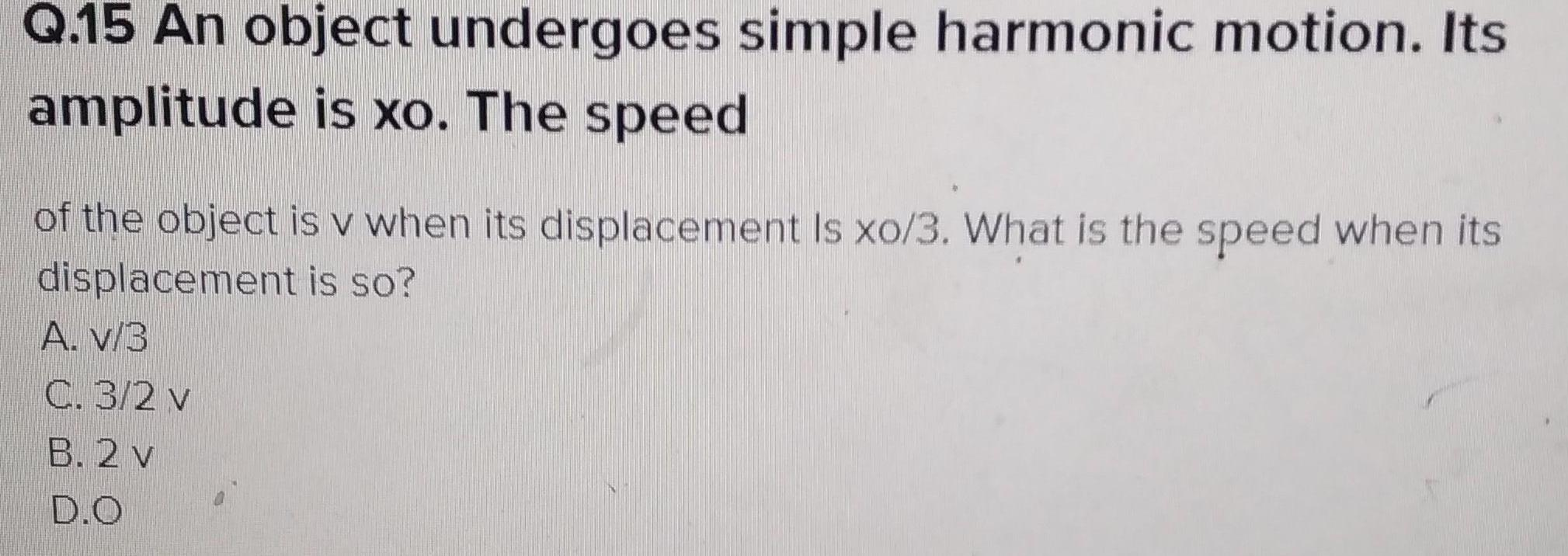Physics
Simple harmonic motion
Q 15 An object undergoes simple harmonic motion Its amplitude is xo The speed of the object is v when its displacement Is xo 3 What is the speed when its displacement is so A V 3 C 3 2 v B 2 v D O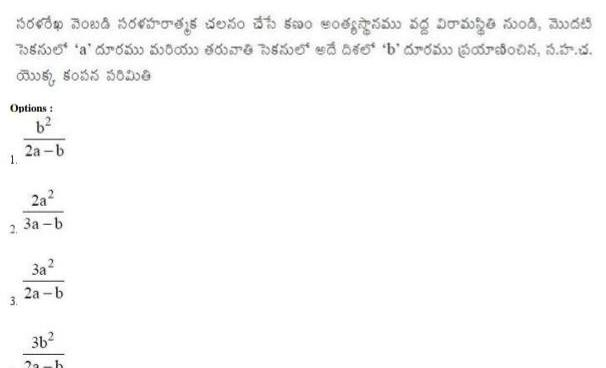Physics
Simple harmonic motion
Sop Boud oss doo h 500 of 50 borge wood and 5 a 50 50 60 bed so b ordu oro 5 aus 5035 50000 Options b 2a b 2 3 2a 3a b 3a 2a b 36 2 h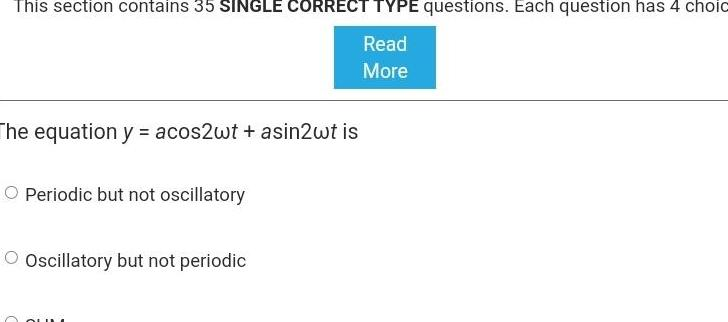Physics
Simple harmonic motion
This section contains 35 SINGLE CORRECT TYPE questions Each question has 4 choic Read More The equation y acos2wt asin2wt is O Periodic but not oscillatory O Oscillatory but not periodic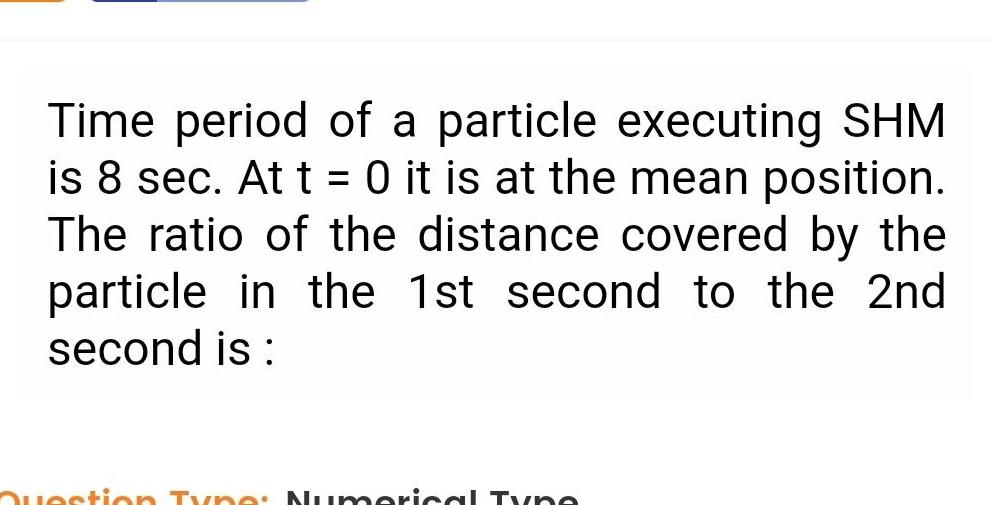Physics
Simple harmonic motion
Time period of a particle executing SHM is 8 sec At t 0 it is at the mean position The ratio of the distance covered by the particle in the 1st second to the 2nd second is Type Nu perical T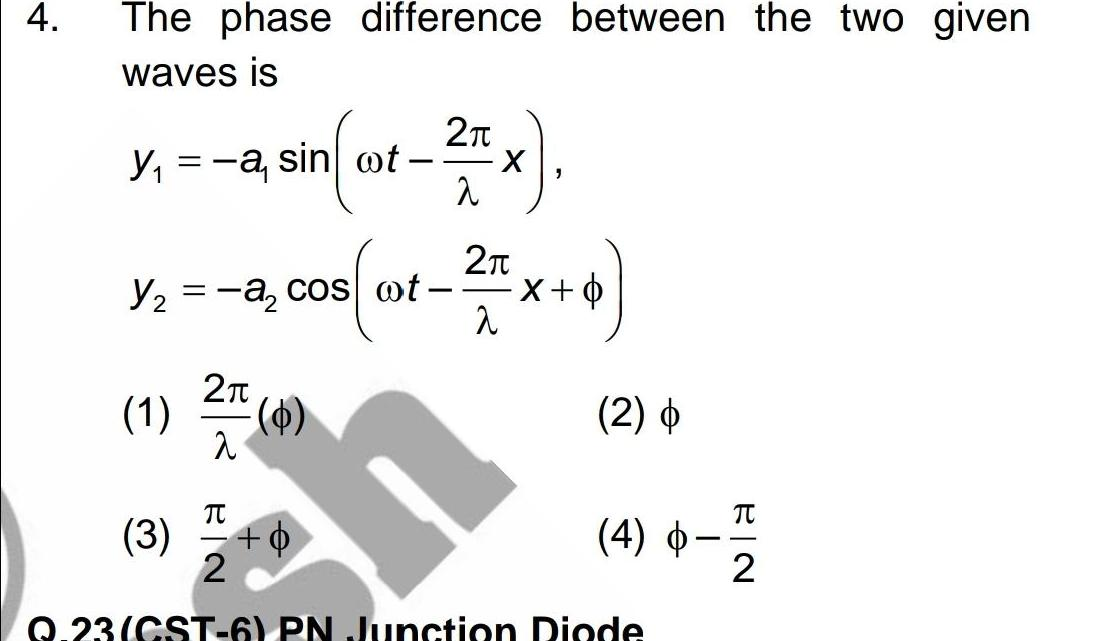Physics
Simple harmonic motion
4 The phase difference between the two given waves is Y a sin ot 2 1 2 Y a cos ot 3 0 2 2 a X 2 x 4 2 4 Q 23 CST 6 PN Junction Diode TU 2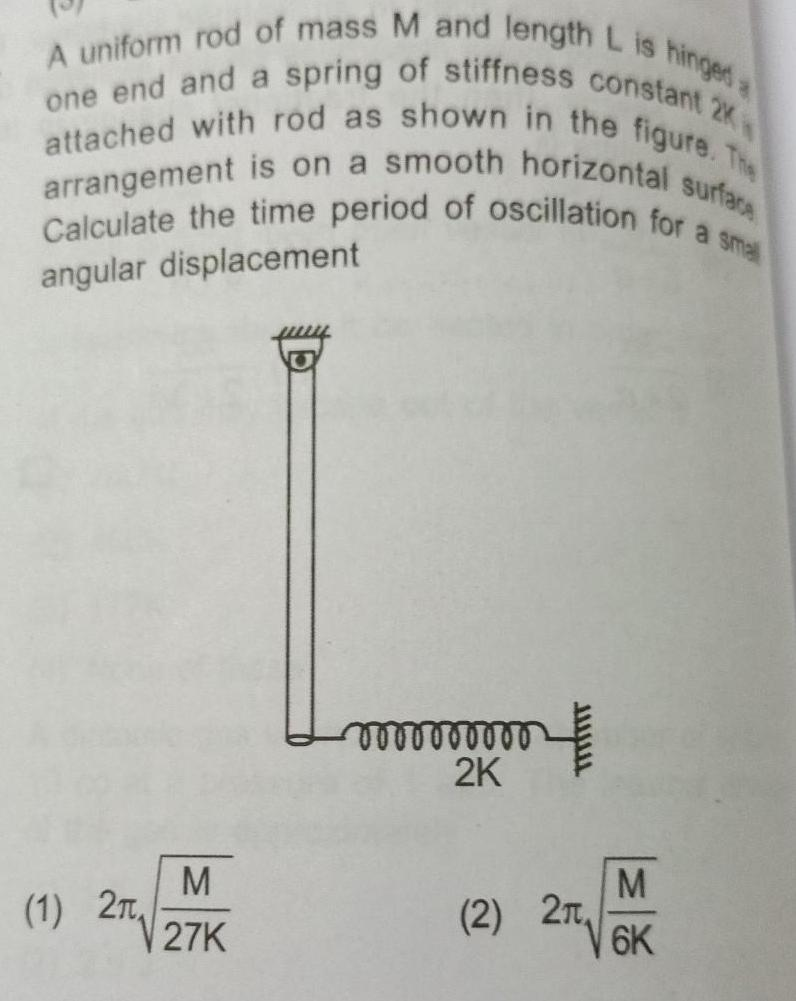Physics
Simple harmonic motion
A uniform rod of mass M and length L is hinged a one end and a spring of stiffness constant 2K attached with rod as shown in the figure The arrangement is on a smooth horizontal surface Calculate the time period of oscillation for a small angular displacement M 1 2 27K mmmm 2K 2 2 M 6K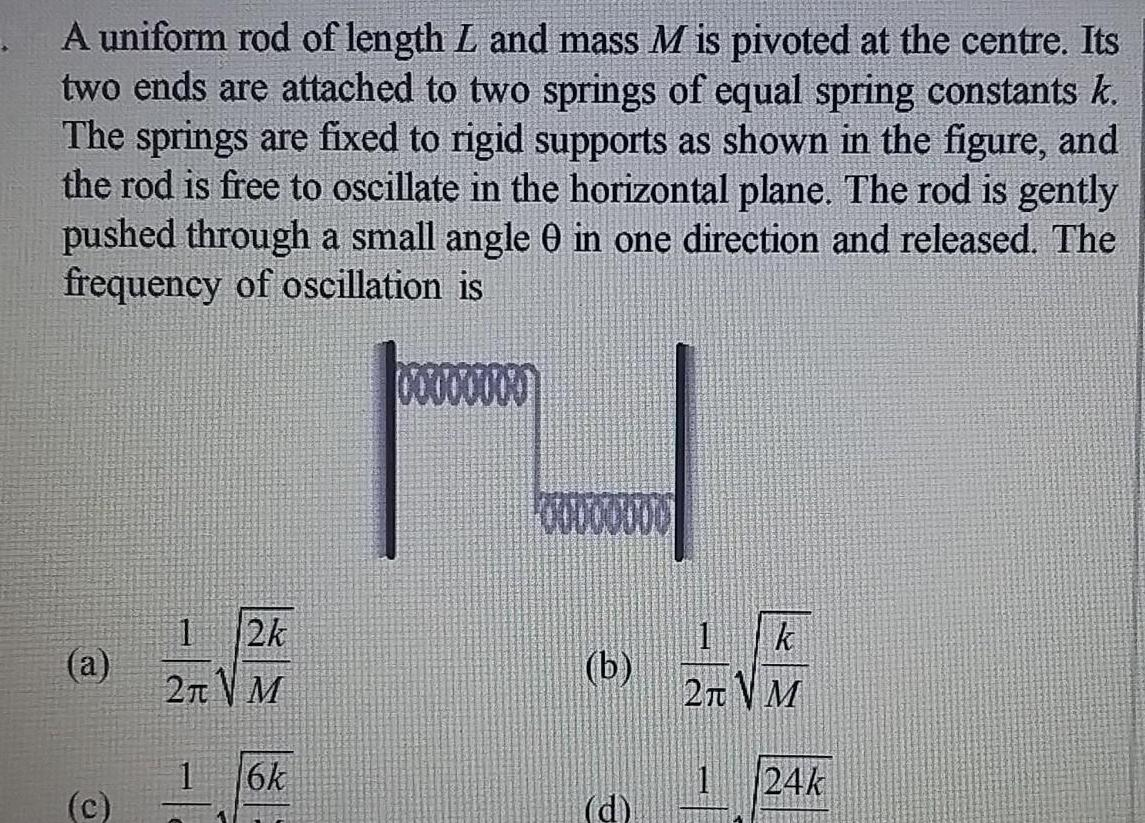Physics
Simple harmonic motion
A uniform rod of length L and mass M is pivoted at the centre Its two ends are attached to two springs of equal spring constants k The springs are fixed to rigid supports as shown in the figure and the rod is free to oscillate in the horizontal plane The rod is gently pushed through a small angle 0 in one direction and released The frequency of oscillation is a c 1 12k 2TM 1 6k b d k 2TM 24k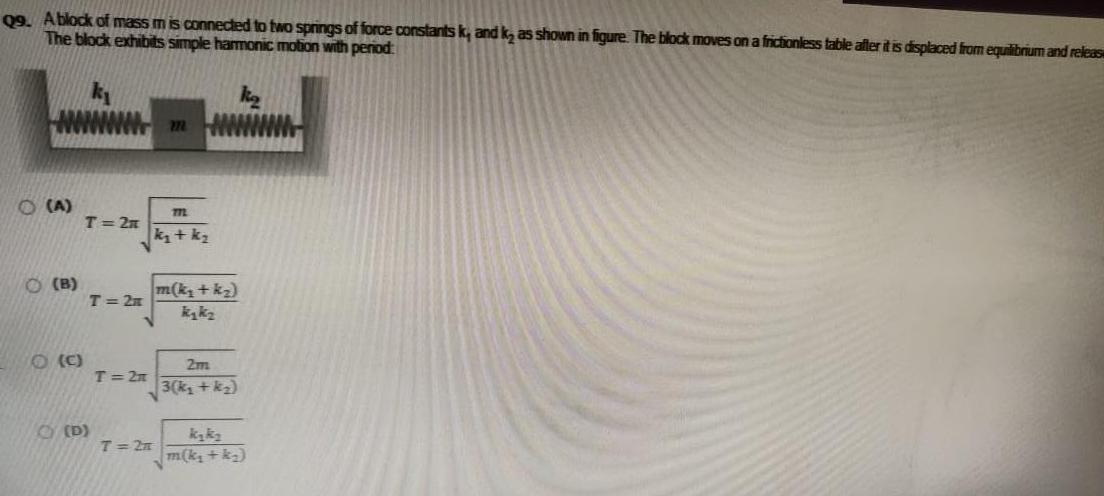Physics
Simple harmonic motion
Q9 A block of mass mis connected to two springs of force constants k and k as shown in figure The block moves on a frictionless table after it is displaced from equilibrium and releas The block exhibits simple harmonic motion with period A O B O C T 2n T 2n D T 2n m 772 k kq T 2n m k k kykz 2m 3 k k kyky m k k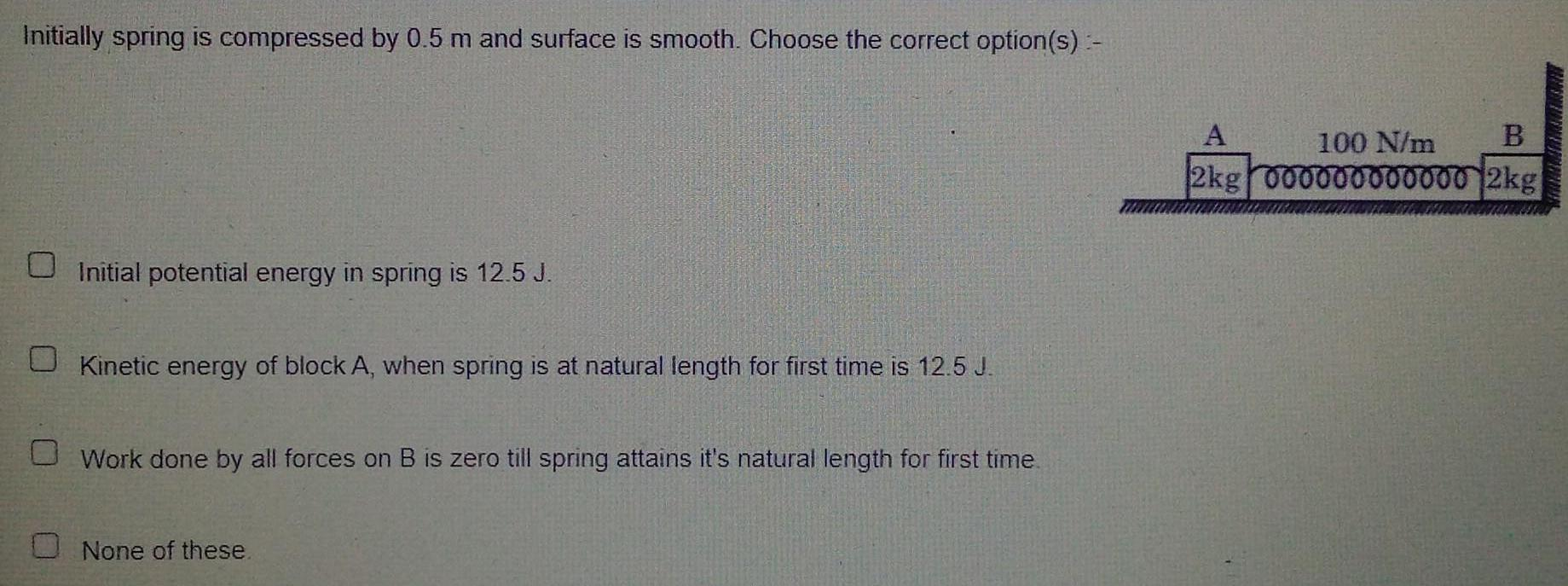Physics
Simple harmonic motion
Initially spring is compressed by 0 5 m and surface is smooth Choose the correct option s Initial potential energy in spring is 12 5 J Kinetic energy of block A when spring is at natural length for first time is 12 5 J Work done by all forces on B is zero till spring attains it s natural length for first time None of these A B 100 N m 2kg 000000000000 2kg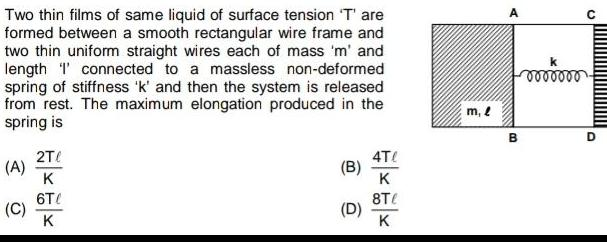Physics
Simple harmonic motion
Two thin films of same liquid of surface tension T are formed between a smooth rectangular wire frame and two thin uniform straight wires each of mass m and length I connected to a massless non deformed spring of stiffness k and then the system is released from rest The maximum elongation produced in the spring is A C 2T K 6T K B D 4T K 8T K m l A B mmm D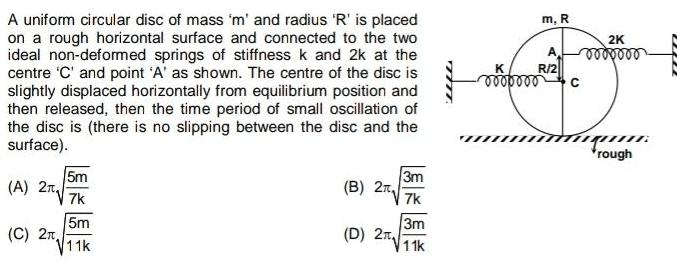Physics
Simple harmonic motion
A uniform circular disc of mass m and radius R is placed on a rough horizontal surface and connected to the two ideal non deformed springs of stiffness k and 2k at the centre C and point A as shown The centre of the disc is slightly displaced horizontally from equilibrium position and then released then the time period of small oscillation of the disc is there is no slipping between the disc and the surface A 2 C 2 5m 7k 5m 11k B 2 D 2 3m 7k 3m 11k m R R 2 ooooooo C 2K 0000000 qu rough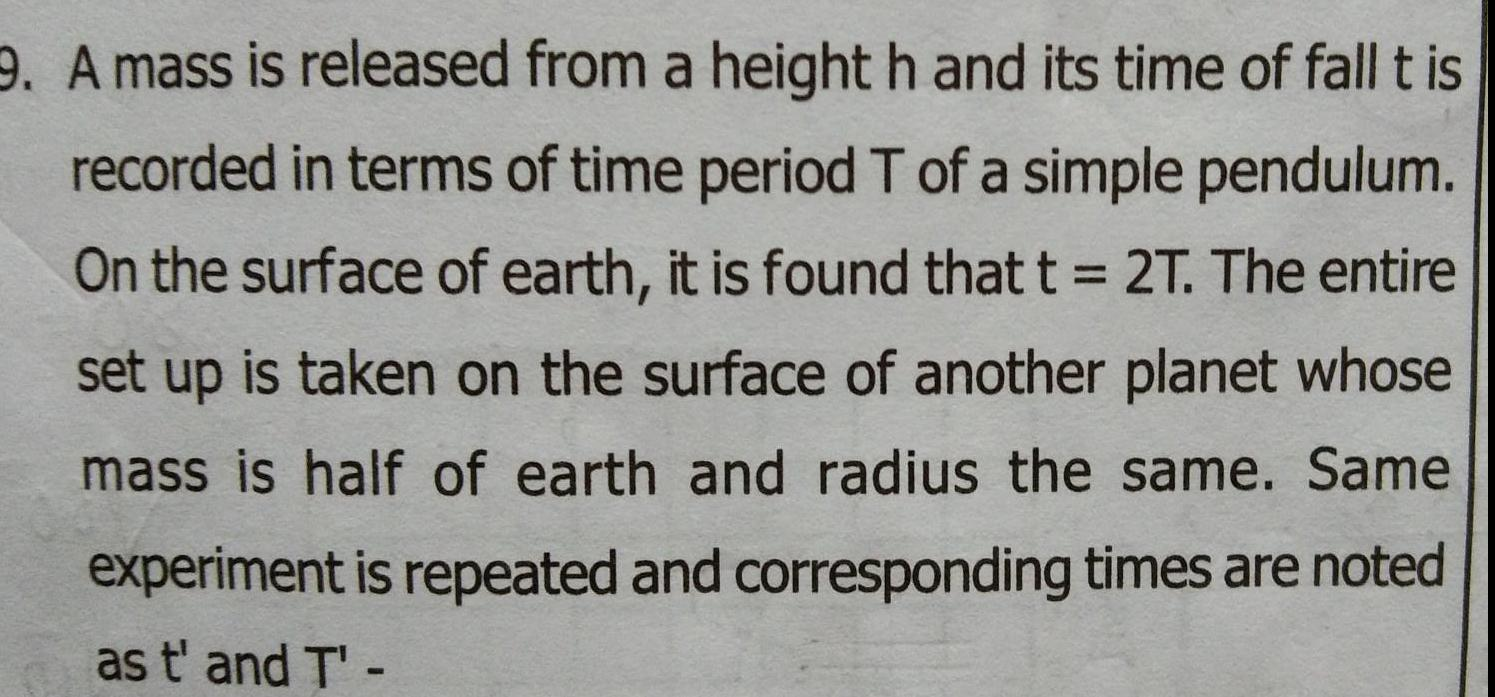Physics
Simple harmonic motion
9 A mass is released from a height h and its time of fall t is recorded in terms of time period T of a simple pendulum On the surface of earth it is found that t 2T The entire set up is taken on the surface of another planet whose mass is half of earth and radius the same Same experiment is repeated and corresponding times are noted as t and T